## Introduction

Decision making under risk and uncertainty is a topic of inquiry in disciplines as diverse as psychology, economics and biology1,2,3,4. Although different in several aspects, these different disciplinary accounts all assume that the likelihood of choosing a risky option is affected by the variability of the option's possible outcomes. To test how risk sensitive people are, subjects are usually presented with a monetary gamble where there is a safe choice and a risky choice. For example, the safe choice rewards the subject with a fixed payoff P with 100% certainty, whereas the risky choice rewards the subject with a higher payoff Q (Q > P) only half of the time. In the equivalent mean payoff gamble5, the rewards are designed such that the mean payoff of the safe and the risky action is the same. Because both options have the same mean payoff, no action should be preferred. However, when people are faced with dicey decisions, a well-documented trend holds6,7,8: If the stakes are sufficiently high, people prefer the safe option. They are therefore risk sensitive (risk averse).

The same risk aversion can be observed in animals who, e.g., actively avoid foraging in an area if they cannot reliably find food there9,10. In addition, it has been proposed that animals actively avoid risk due to the increased mortality that risky decisions often entail11. When foraging, animals only take risks when the risk of the decision is outweighed by other factors12,13,14,15. Additionally, foraging animals avoid risk when resources are plentiful, but adopt riskier strategies when resources are scarce16. Further, organisms ranging from bacteria17 to birds18 are suggested to mitigate risk in their reproductive success via bet hedging19,20,21,22,23,24. However, in these natural situations risky behavior often does not compensate for the potential cost of taking the risk. Thus, while these circumstances could explain the evolutionary advantage of risk sensitive behavior in many natural settings and species, they do not offer a clear rationale for why humans would be prone to avoiding the risky action in the equivalent mean payoff gamble where the average payoff of each choice is the same.

Nevertheless one should assume that the risk sensitivity of both humans and animals may be shaped by a common fundamental principle25. Moreover, there is considerable evidence from cognitive neuroscience that loss aversion has a neural basis26. Neurophysiological measurements suggests that different regions of the brain process value and risk assessment27,28. This work also implies that the neural circuitry that encodes risk sensitivity (or its building blocks such as loss aversion) is phylogenetically ancient. However, the origin of this neurally encoded risk sensitivity is rarely discussed (but see refs. 11, 29, 30), even though there is strong evidence that risk-taking behavior has significant genetic components31,32.

This poses a puzzling question: how can evolution select for risk sensitivity in the equivalent mean payoff gamble if no choice has a higher mean payoff (fitness)?

## This is not bet hedging

In contrast to the equivalent mean payoff gamble studied here, it has been shown33,34,35 that if the risky action comes with a higher payoff than the certain one, evolution will favor risk seeking behavior. An alternative explanation might be bet hedging, where individuals optimize their fitness by sacrificing their mean fitness to decrease variation on fitness. While geometric mean fitness maximization or bet hedging can influence the payoffs received36 and thus contribute to the evolution of risk sensitivity, these strategies do not affect the payoff in the equivalent mean payoff gamble. Geometric mean fitness maximization and bet hedging requires multiple games, which is not allowed in the gamble studied here. One might argue that not the individual but the population itself is heterogeneous and thus as a whole hedges the bets. This, however, would require selection to occur on the population level, which does not explain risk sensitivity in individuals.

## Prospect and expected utility theory

First, the classical economists' account of risk sensitivity is in terms of the shape of the utility function in expected utility theory. Specifically, the curvature of the utility function is interpreted to measure the agent's risk attitude and the more concave the utility function, the more risk averse the agent will be. A concave utility function corresponds to the notion of diminishing marginal utility of wealth according to which “the additional benefit which a person derives from a given increase of his stock of a thing, diminishes with every increase in the stock that he already has” [Ref. 37, p. 79]. Second, cumulative prospect theory, perhaps the most influential descriptive account of decision making under risk in psychology and behavioral economics, models risk aversion in terms of three different but related concepts: diminishing marginal utility, loss aversion (i.e., the pain of losses is felt stronger than the joy of equivalent gains) and probability weighting (i.e., the elevation of the weighting function and thus the degree of over/underweighting of small probabilities of gains and losses, respectively)3,38. While both theories describe the human preference, it is not clear why such behavior has an evolutionary advantage in the equivalent mean payoff gamble.

## External factors

Of course, the gamble described above is a crude simplification of human choice, which can be shaped by many other factors. External factors such as framing39, how the odds are presented40, or if the decision has to be made from experience or from description41 play a role in human decision making. The relative value of the payoff to the subject as well as whether the gamble is real or hypothetical can have an effect on the subject's preference42. Internal factors such as age43,44, cognitive ability45 and habits or personal circumstance46,47 influence the subject's decision, as well as how the subject weighs the potential value of losses and gains3. Without downplaying the importance of these factors, the question remains: Where did this risk sensitive behavior originate from?

## Small population size and risk sensitivity

Previous studies have reported that in small populations of evolving organisms, the fitness of riskier behaviors is significantly affected by the variance in the payoff of the behavior19,48. This observation suggests that strategies that minimize the variance in the payoff of a gamble should have a selective advantage only in small populations. Consequently, evolution in small populations could potentially explain the origin of risk sensitivity by humans in equivalent mean payoff gambles.

In order to study the evolution of the strategy, we simulate a population of agents whose choice of strategy is determined genetically and inherited by the agent's offspring. The payoff that the agent receives is taken as the agent's fitness. A small probability of mutation introduces variation, so that alternative strategies from the ancestral one can be explored. Each agent makes only one decision during its lifetime that determines its fitness, which means that the agents are potentially making a life (positive payoff 1/χ) or death (zero payoff) decision. Such a life or death decision is akin to a rare lifetime event that has a large impact on an individual's fitness, such as mating and mate competition49. We use this agent-based simulation to explore how small a single population has to be in order to have a significant impact on the evolution of risk sensitivity. Additionally, we implement an island-based model to test whether larger populations that were segmented into small groups (with the possibility of migration between groups) could still select for the evolution of risk sensitivity. Although this model cannot take into account the complexity of human evolution nor the exact circumstances thereof, it can address the plausibility of risk sensitivity as an evolutionary adaptation to equivalent mean payoff gambles due to small population sizes.

## Results

### Theory of selection for variance

The tendency for natural selection to select against variance in offspring number has been discussed before. Indeed, Gillespie has argued that large variance in offspring number could be selected against because adverse outcomes (few or zero offspring) cannot easily be balanced by favorable outcomes (large broods) for individuals of the same species, because the individuals without offspring may not get to try the “offspring lottery” again19,48. Further, Gillespie proposed an approximate mean actual fitness that takes the variance in the offspring number distribution into account (see Equation ).

To test whether evolution can explain the emergence of risk sensitive strategies, we generalize the equivalent mean payoff gamble so that there are an infinite number of possible choices, parameterized by the probability χ to obtain the high payoff. We choose this payoff to be 1/χ, so that the mean payoff of any choice will be 1. We will call any of the possible gambles a strategy and denote each strategy by the probability χ. The choice χ = 1 then implies that the agent chooses the safest gamble. In this game, there is no limit on how risky the gamble is, except we do not permit strategies with χ = 0, as they are not normalizable.

Gillespie's fitness estimate wact strongly depends on the strategy choice χ (because it determines the variance in the offspring number distribution) as well as population size (Figure 1A). To illustrate the phenomenon, imagine one risky strategy (χ = 0.01) and 99 non-risky strategies (χ = 1.0). The risky strategy wins on average in one out of hundred gambles and receives a payoff of 100. While the one lucky gamble makes it 100 times fitter than all others, this win happens rarely. However, in the small population of 100 organisms, the risky strategy will have 0.0 payoff and no offspring in 99 out of 100 gambles (generations), it will thus die out more likely. Only in an infinite population will this risky strategy never die out. Therefore, small populations favor, risk averse strategies.

Thus, this theory explains why agents that evolved in small populations show a preference for risk sensitive strategies, whereas agents that evolved in larger populations showed no such strategy preference. We can test the theory directly by measuring the probability Π (the fixation probability) that a perfectly risk-sensitive strategy (χ = 1) can invade (and replace) a homogeneous population consisting of strategies with choice χ ≤ 1. We find that the observed probability of fixation (shown in Figure 1B for a population size of N = 50) agrees qualitatively with the fixation probability calculated using Gillespie's fitness in Kimura's formula (dashed line in Figure 1B), but not quantitatively. Indeed, an effective fitness of about half of Gillespie's estimate reproduces the simulations almost exactly, which corroborates earlier findings22). Since our computational model does otherwise not produce controversial results (see below), we suggest that Equation (3) is quantitatively wrong and therefore does not represent our model.

### Evolution in a single population

Evolution is not explained by fixation probabilities alone. In nature we find populations containing a range of mutated strategies that adapt in a heterogeneous background of other mutants. Instead of relying on fixation probabilities alone, we now investigate how in an agent-based model strategies playing the equivalent mean payoff gamble evolve, assuming a non-zero mutation rate. This is particularly important because very risky strategies, in case they win, will have very many offspring in that generation, which all have the chance to mutate.

Each agent in a population is represented by a single probability χ (the agent's inherited gambling strategy), where χ determines the fitness of the agent. Every agent only plays the gamble once in their lifetime, so their fitness is determined by polling a random variable X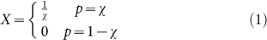exactly once, where p is the probability to receive the corresponding payoff and χ is the agent's strategy. An agent equipped with a strategy χ > 0.5 is considered risk sensitive, whereas an agent with a strategy χ < 0.5 is considered risk-prone. All else being equal, we expect that evolution should not prefer any strategy over another, because the mean payoff of a species (individuals with the same χ) should be the same regardless of χ.

If population size does not have a significant effect on the evolution of risk sensitivity, we would expect the strategy preference of any individual to drift neutrally, so that at the end of the evolutionary run (generation 950) the expected mean population strategy is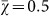(the mean of a uniformly distributed random variable constrained between zero and one). Instead, we observe in Figure 2A that the mean χ converges to 0.6941 ± 0.0139 (mean ± two standard errors).

Similarly, we would expect that if the strategy drifts neutrally, we should observe χ to be distributed in a uniform manner at the end of the evolutionary runs. Instead, for a population size of N = 100, we observe in Figure 2B a distribution that departs significantly from uniformity (Wilcoxon rank sum test P = 7.795410−22 between this distribution and a uniform random distribution). This result suggests that population size plays a critical role in shaping what strategies evolve in the agent population.

To further explore the effect of population size on evolved strategy preference, we ran the evolutionary simulation with different fixed population sizes. Figure 3 demonstrates that the final evolved strategy depends strongly on the population size. These results highlight that agents in smaller populations prefer risk sensitive strategies that receive a lower payoff but with higher reliability. In contrast, agents in larger populations do not show a preference for risk sensitivity nor risk-seeking strategies and converge on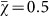because all strategies perform roughly the same in large populations, that is, the χ of individual strategies drifts neutrally.

Throughout evolutionary history, humans have experienced at least two population bottlenecks that reduced the human population to as few as 1,000 individuals50,51. However, a population size of 1,000 individuals is unlikely to be small enough to evolve risk sensitive behavior as a dominant strategy in the population19,48. Instead, a more likely explanation is that humans have lived in groups of about 150 individuals throughout their evolutionary history52,53, which plausibly could have been a small enough effective population size for risk sensitivity to have evolved.

### Evolution in groups

In the previous section, we demonstrated that agents in small populations evolve a preference for strategies with low variance in their payoff distribution, i.e., risk sensitivity. The group size for humans throughout evolutionary history has been proposed to be around 150 individuals52,53, which suggests that evolving in such small groups could have been the reason behind the evolution of human risk sensitivity. However, a small group size and a small population size are two different things. While humans might have lived in small groups of 150 individuals, the total population size of humans has been much larger and were only at times as low as 1,000 individuals50,51. Even though selection may occur within groups of about 150, individuals likely migrated between groups. Migration could have caused selection to effectively act on much larger groups (or even the entire human population) negating the selection for a variance effect.

We can simulate such an environment using an island-based evolutionary model (see Methods), in which individuals live in groups (the “islands”) that randomly exchange individuals with each other via migration. For example, we can run 1,000 replicate evolutionary experiments with 128 groups of 128 individuals each, with varying migration rates. In this configuration the total population size is 16,384 individuals, which according to Figure 3 should result in agents evolving no strategy preference. Figure 4 shows that regardless of the migration rate, the group size and not the total population size determines whether agents evolve risk sensitive strategies. This result suggests that even with migration between groups, the effective population size that selection acts on is determined by the group size and not the total population size. While this result might seem surprising, one has to take into account that selection happens for each group separately and these virtual agents only compete against agents within their group and not against everyone in the entire population, which explains why the migration rate does not affect the evolution of risk aversion in this model.

When we change the size of the groups but fix the total population size (i.e., increase the group size and reduce the number of groups) while keeping the migration rate at a constant 0.1, we again observe that the group size critically determines the preferred evolved agent strategies (Figure 5). Risk sensitive strategies are preferred in smaller groups and no strategy is preferred in larger groups. This result recapitulates the results from Figure 3, which shows that the preference for strategies with low payoff variation (i.e., risk sensitivity) depends on the effective population size.

### Relative value of the gamble

Another way to alter risk sensitivity in humans is by changing the relative value of the payoff42. When the gamble is about small amounts of money (i.e., “peanuts” gambles or hypothetical money), humans tend to be less risk averse, whereas raising the relative value of the gamble increases risk aversion. In our evolutionary simulation, agents play the gamble a single time and the payoff they receive is their only source of fitness. This constraint effectively turns the gamble into a life or death situation, similar to a game with extraordinarily high stakes.

To simulate lower-stakes gambles, we add a baseline payoff (β) to the payoff so that the fitness of the agent becomes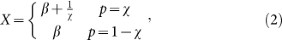where p is the probability to receive the corresponding payoff and χ is the agent's strategy. Typical gambles humans partake in fall either in the loss or in the gain domain. In biological systems, on the other hand, organisms accumulate resources in order to ultimately produce offspring. The “gambles” these organisms undertake will influence the number of offspring, which will be positive or zero. Thus, we cannot differentiate between losses or gains in the same way people conceive a gamble for money. Therefore, gains and losses must be considered relative to fitness.

When we run the evolutionary simulation with a population size N = 100 for various values of β, we observe that the larger the baseline β becomes, the more often strategies return to an unbiased choice (Figure 6). This result is expected because fitness differences only matter if their relative impact is larger than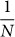54,55. Thus, risk sensitive strategies will only be selected for when the outcome of the gamble represents a significant portion of the individual's fitness when taking the population size into account.

### Repetition of the gamble

Thus far, we have only investigated one-time gambles. What happens when the agents engage in the same gamble multiple times during their lifetime? On average, repeating the gamble reduces the variance in the overall payoff the agents receive and if games are played infinitely, then the payoffs will converge to the same mean. In this experiment, we do not consider situations where agents can change their behavior based on previous experiences56, but rather focus on unconditional responses. We observe that the agents no longer evolve a preference for risk sensitivity if the gamble is repeated several times in a lifetime (Figure 7). At the same time, the effect of repetition depends strongly on the population size, such that smaller populations still evolve risk sensitive strategies with as many as 10 repetitions of the gamble. Therefore, a preference for risk sensitivity will only evolve for those gambles that are encountered a few times during an individual's lifetime.

## Discussion

We hypothesized that risk sensitivity in humans could have been an evolutionary adaptation to living in small groups. We tested this hypothesis by evolving artificial agents whose fitness is determined by a single choice during their lifetime in groups of varying size and where that choice is encoded genetically and thus heritable. We observed that a preference for risk sensitivity does indeed evolve, but only when the group size is sufficiently small. However, our results differ quantitatively from those predicted19. While this has been suggested before22, this has not been tested using agent based modeling. New in our study is the introduction of a mutation rate that allows strategies to change over evolutionary time scales. Based on this we find the following novel results: Even with mutations, small populations are still causing risk aversion to evolve, the relative value of the gamble still matters and as expected, repeating the game has drastic effects on the payoff variance.

Without a mutation rate we would expect a single strategy as the winner, instead we find a distribution of strategies in the population, which is not predictable from a zero mutation rate assumption. These findings align with reports from earlier work that humans lived in groups of about 150 individuals for a large portion of their evolutionary history52,53, providing a plausible evolutionary explanation for the risk averse behavior commonly observed in humans. In other words, these findings provide a quantitative foundation to the idea that evolution can explain risk sensitivity29. The computational model inevitably abstracts many nuances of human evolution. Because of this, however, we are able to show that all organisms who experienced similar situations could, in theory, have evolved risk aversion.

Additionally, we find that risk sensitivity is the preferred evolutionary adaptation to life in small groups when these groups are embedded within much larger groups, even with a large amount of migration between groups. However, it is important that the risky decisions occur only rarely during an individual's lifetime and where the outcome of the gamble represents a significant effect on the individual's fitness. If the gamble has a negligible impact on fitness (e.g., only small gains are at stake) or if the risk is encountered regularly in the individual's lifetime, then the selective advantage of risk sensitivity will be lost. Examples of such rare, high-risk, high-payoff gambles include mating and mate competition49.

Our work does not imply that no risk-seeking strategies can possibly evolve. What we show is that risk sensitivity evolves on average, but the distribution of strategies within a population is quite broad (Figure 2). Thus, while on average agents are risk-averse if they evolve in a small population, there will always be some agents that are extremely risk-seeking. Such agents can do extraordinarily well by chance and persist, but their genes are ultimately destined for extinction.

While our model is only haploid and uses a single locus, we do not expect a diploid model using multiple loci to have qualitatively different results from those presented in this paper. Regardless, gene flow in diploid organisms in an island model and its impact on the evolution of risk sensitivity is likely an interesting extension of this experiment to pursue in future work.

## Methods

### Single population evolutionary model

We use a genetic algorithm applied on a population of agents to simulate evolution of the population57. Each agent in this population is defined by a probability χ (the “choice”), which encodes the agent's strategy. We seed the initial agent population by assigning every agent a random χ drawn from a uniform distribution (0, 1] with a variance of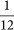. Varying the initial starting condition has no significant effect on the outcome of the experiments. Every agent in the population only plays the gamble once in its lifetime to determine its fitness, where χ is the probability to receive a fitness of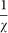or receive 0.0 fitness with a chance 1 − χ. The strategy of each agent can only change due to evolution, i.e., strategies cannot change during the agent's lifetime.

Once all of the agent fitnesses are evaluated for a given generation, the agents produce offspring into the next generation in proportion to their fitness, i.e., we use fitness proportional roulette wheel selection to determine the next generation of individuals58, implementing the Wright-Fisher process. Offspring inherit the strategy χ from their parent (no sexual recombination), except that 1% of all offspring are subjected to mutation. If an offspring is subject to mutation, its new strategy is drawn randomly from a uniform distribution (0, 1]. We repeat this evolutionary process every generation with a fixed population size for 1,000 generations.

### Theory of selection for variance in offspring number

Gillespie suggested that in finite populations where the fitness of individuals carries a stochastic component (modeled by a mean μ and a variance σ2), the actual realized fitness wact is given by19,48: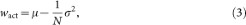where N is the population size. Because in the equivalent mean payoff gamble agents receive a payoff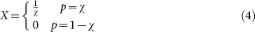the variance becomes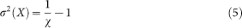and the actual fitness of a strategy χ is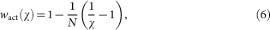as the mean of X in Equation (4) (in an infinite population) equals 1. The fitness advantage s of a strategy with χ = 1 versus a strategy χ is then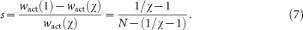We use Equation (6) to compute the actual fitness of a strategy using a given χ while taking the size of the population N into account (Figure 1A) and we use the fitness advantage (7) in the calculation of the fixation probability using Kimura's formula in Figure 1B.

### Island-based evolutionary model

In our second set of experiments, we use an island-based evolutionary model to simulate an environment in which thousands of individuals are evolving in several small groups. For an overview of island models and the effect of population size, see Refs. 59, 60. Island models have three parameters: The size of a single group, the number of groups and a migration rate defining how many individuals per group are moved randomly to new groups during each generation. If an agent is selected to migrate, we randomly select a new group and a random agent within that group and switch agents. Thus, our island-based evolutionary model implements several single population evolutionary models with a fixed fraction of individuals migrating between the populations every generation. The migration rate is the probability that an agent will be picked for migration per generation. For example, a migration rate of 0.1 implies that 10% of the agents in the entire population are picked to switch (affecting up to 20% of the population, as each switch affects two agents).

Typically, island models are used to speed up evolution in rugged fitness landscapes and increase genetic diversity within the population. In this experiment, we are not concerned with ruggedness nor diversity. Instead, we use an island model because it best approximates the scenario of individuals evolving in multiple small groups with some level of inter-group migration.

### Retracing the line of descent

At the end of each evolutionary run, we reconstruct the line of descent (LOD) by picking a random agent in the population and tracing back to the first generation using only direct ancestors61. This procedure rapidly converges on the last most recent common ancestor (LMRCA) that swept the population. In our experiments, we determined that the agents on the LOD at generation 950 most often represented the LMRCA, thus we used those agents as the final representative agent for their respective evolutionary run. The LOD between the first agent and the LMRCA of a population contains all mutations that fixed during evolution, while all other mutants were outcompeted. Thus, analyzing an evolutionary run's LOD enables us to retrace the evolutionary history of the population.